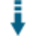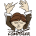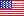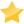# How to Implement PHP Type Safety Using Ristretto PHP: Manipulate values in type safe way using classesDownload .zip
 InfoDocumentationView files (12)Download .zip Reputation Support forum Blog (1) Links
 Ratings Unique User Downloads Download Rankings Not yet rated by the users Total: 33 All time: 10,884 This week: 200Version License PHP version Categories ristretto-php 1.0.0 MIT/X Consortium ... 5 PHP 5, Cryptography, Data types
Description

Author

This package can manipulate values in type safe way using classes.

It implements a base class named Ristretto that implements essential functions like comparison of values of the same type and hexadecimal encoding of a value.

The package also provides sub-classes that implement specific functions of some data types, like generating random values, value hashing, and arithmetic operations.

Currently, it provides the following data type classes:

- Group element

- Public Key

- Scalar Value

- Secret Key

Innovation AwardNovember 2022 Number 6 Type safety is a characteristic of languages that require certain operations with values of the correct values. This characteristic is good for catching bugs early in application development. PHP was not originally a type-safe language. PHP is more tolerant of developer mistakes. This fact makes PHP a more accepted language among developers without formal education in programming. This package provides a solution to make PHP more type-safe to avoid that applications shipping code with programming mistakes. It provides several classes that implement type-safe operations, including some that are useful for cryptography applications. With this package, you can implement cryptography applications with greater quality when compared to applications that do not use type safety. Manuel LemosPerformance Level
Name: Classes: Scott Arciszewski `` 36 packages by Scott ArciszewskiUnited States ??? 1188 171 in United States9612 in United StatesLevel 1Innovation awardNominee: 28x

Winner: 1x

# Ristretto (PHP)

Implements a type-safe API for working with the Ristretto Group in PHP projects.

## Installing

``````composer require paragonie/ristretto
``````

## Documentation

There are two basic types: `ScalarValue` and `GroupElement`.

The `ScalarValue` object wraps a big integer between 0 and the order of the Ristretto Group, `L`.

The `GroupElement` object wraps a group element of the Ristretto Group.

If an analogy helps, in the world of Ed25519 and X25519, the `ScalarValue` is your secret key, and `GroupElement` is your public key.

For that reason, there are also a `SecretKey` and `PublicKey` class, which contains some basic helper methods for ease-of-use.

## Usage

You can convert from scalars to group elements with `multBase()`, and then use `scalarPointMultiply()` to perform a commutative group action (e.g. Diffie-Hellman).

``````<?php
use ParagonIE\Ristretto\{GroupElement, ScalarValue};

\$aliceSecret = ScalarValue::random();
\$alicePublic = \$aliceSecret->multBase();
\$bobSecret = ScalarValue::random();
\$bobPublic = \$bobSecret->multBase();

// You can perform a similar commutative group action
\$aliceToBob = \$aliceSecret->scalarPointMultiply(\$bobPublic);
\$bobToAlice = \$bobSecret->scalarPointMultiply(\$alicePublic);
var_dump(\$aliceToBob->equals(\$bobToAlice)); // bool(true)
``````

Otherwise, most operations are within a given type (GroupElement to GroupElement, ScalarValue to ScalarValue).

### GroupElement

``````<?php
use ParagonIE\Ristretto\{GroupElement};

\$x = GroupElement::random();
\$y = GroupElement::random();

\$w = \$z->sub(\$y);
var_dump(\$w->equals(\$x)); // bool(true)
``````

## Example

This is a PHP implementation of the libsodium example protocol.

> Perform a secure two-party computation of `f(x) = p(x)^k`. `x` is the input sent to the second party > by the first party after blinding it using a random invertible scalar `r`, and `k` is a secret key > only known by the second party. `p(x)` is a hash-to-group function.

``````<?php
use ParagonIE\Ristretto\{GroupElement};

// -------- First party -------- Send blinded p(x)
\$x = random_bytes(64);

// Compute px = p(x), a group element derived from x
\$px = GroupElement::fromHash(\$x);

// Compute a = p(x) * g^r
\$r = ScalarValue::random();
\$gr = \$r->multBase();

// -------- Second party -------- Send g^k and a^k
\$k = ScalarValue::random();

// Compute v = g^k
\$v = \$k->multBase();

// Compute b = a^k
\$b = \$k->scalarPointMultiply(\$a);

// -------- First party -------- Unblind f(x)
// Compute vir = v^(-r)
\$ir = \$r->negate();
\$vir = \$v->scalarPointMultiply(\$ir);

// Compute f(x) = b v^(-r) = (p(x) g^r)^k * (g^k)^(-r)
//              = (p(x) g)^k g^(-k) = p(x)^k

// --------- Correctness testing -----------
// If you knew both p(x) and k, you could calculate it directly.

// Directly calculate p(x)^k with both parties' secrets
\$pxk = \$px->scalarPointMultiply(\$k);
var_dump(\$fx->equals(\$pxk)); // bool(true)
``````Files
File Role Description.github (1 directory)src (5 files)tests (1 file)composer.json Data Auxiliary dataphpunit.xml Data Auxiliary datapsalm.xml Data Auxiliary dataREADME.md Doc. Read meFiles / .github
File Role Descriptionworkflows (2 files)Files / .github / workflows
File Role Descriptionci.yml Data Auxiliary datapsalm.yml Data Auxiliary dataFiles / src
File Role DescriptionGroupElement.php Class Class sourcePublicKey.php Class Class sourceRistretto.php Class Class sourceScalarValue.php Class Class sourceSecretKey.php Class Class sourceFiles / tests
File Role DescriptionRistrettoTest.php Class Class source

 All time: 10,884 This week: 200About usAdvertise on this siteSite mapNewsletterStatisticsSite tipsPrivacy policyContact
For more information send a message to `info at phpclasses dot org`.